## Two large parallel metal plates are 1.6 cm apart and have charges of equal magnitude but opposite signs on their facing surfaces. Take the p

Question

Two large parallel metal plates are 1.6 cm apart and have charges of equal magnitude but opposite signs on their facing surfaces. Take the potential of the negative plate to be zero. If the potential halfway between the plates is then 6.3 V, what is the electric field in the region between the plates

in progress 0
3 months 2021-08-06T09:29:08+00:00 2 Answers 7 views 0

The electric field in the region between the plates is 787.5 V/m

Explanation:

The magnitude of the potential difference between the plates is equal to the product of the magnitude of the electric field and the distance between the plates.

The relationship: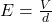Electric field: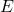is unknown

Voltage supplied: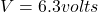distance between the plates: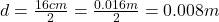Now substitute the values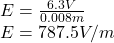The electric field in the region between the plates is 787.5 V/m.

787.5 V/m

Explanation:

the formula to find the electric field in the region between the plates is

E = V/d

where

V=the voltage supplied by the battery (potential)

d=distance between the plates.

E=electric field

Given:

V= 6.3 V

d= 1.6cm/2=> 0.016m/2

E= V/d=> 6.3/(0.016/2)

E= 787.5 V/m

Therefore, the electric field in the region between the plates is 787.5 V/m